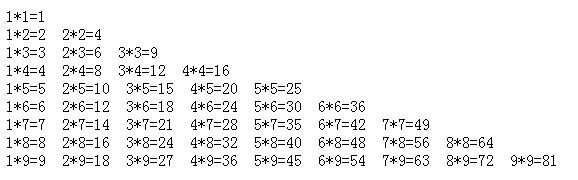# PHP中实现九九乘法口诀表

•```<?php
for(\$i=1;\$i<=9;\$i++){
for(\$j=1;\$j<=\$i;\$j++){
echo \$j,'*',\$i,'=',\$i*\$j,'&nbsp;&nbsp;';
}
echo '<br/>';
}
?>```

```<?php
for(\$i = 1;\$i <= 9; \$i++){
for(\$j = 1;\$j <= \$i; \$j++){
echo \$j."*".\$i."=".\$j*\$i."&nbsp;&nbsp;";
}
echo "<br />";
}
?>```